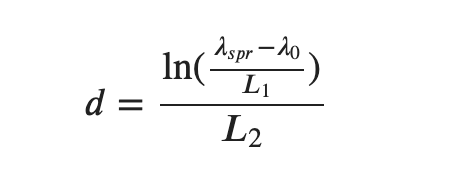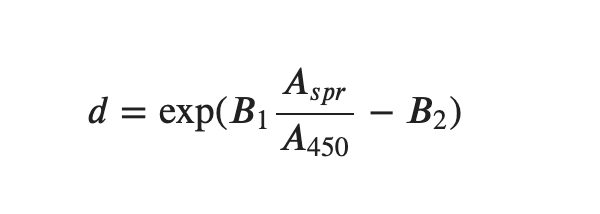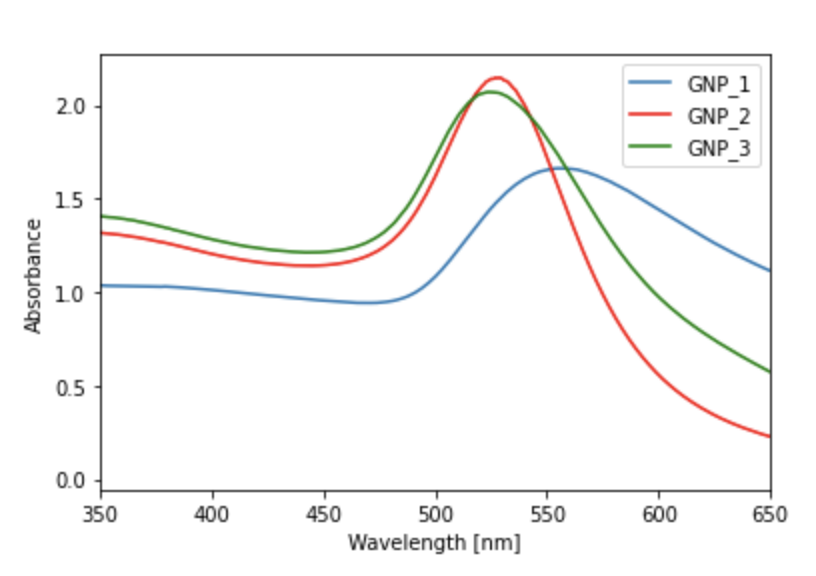# Calculation of Properties of Gold Nanoparticles using Absorbance Data

## This Colab-notebook plots spectra of gold nanoparticles from SpectroWorks™. The peak surface plasmon resonance (SPR) is calculated and this can be used to find an estimate for the diameter of the particles, amongst other properties.

Metal nanoparticles have a multitude of uses. In particular, gold nanoparticles have been used in biological and medical applications as drug delivery agents, in electronic conductors, in catalysis, sensors and probes.

The optical and electronic properties of gold nanoparticles vary with their physical characteristics, like shape and size.

The diameter of gold nanoparticles are related to their peak surface plasmon resonance (SPR) wavelength. The SPR of a metal particle is the wavelength of light that results in the free electrons on the surface of the metal to oscillate in resonance with the visible light. Generally, the larger the particle size, the larger the SPR.

The peak surface plasmon value can be extracted using UV-VIS Spectrophotometry. Together with experimental parameters, the average diameter of the nanoparticles particles can be estimated. The following equations have been used in literature  to calculate the diameter of gold nanoparticles.Equation 1: λspr is the peak SPR wavelength and λ0, L1, L2 are experimentally determined parameters.Equation 2: Aspr is the absorbance at the peak SPR, A450 is the absorbance at λ = 450nm and B1, B2 are experimentally determined parameters.

This easy to use interactive Colab notebook can analyse hundreds of files of absorbance data in seconds. Currently, we have implemented the following calculations within the notebook:

• Aspr, the absorbance at the peak SPR
• A450, the absorbance at λ = 450nm
• λspr, the wavelength of the peak SPR (usually found within 500 < λ < 600nm)
• Pass/fail tests to confirm whether there is any SPR peak
• Several useful absorption ratios commonly used in gold nanoparticle analysis in addition to the ability to calculate any ratio
• The diameter as given in equation 1 and 2 with adjustable parameters
• Pass/fail tests to confirm whether the data are agreeing with the models defining equation 1 and 2
• The relative width of the peak at 90% of the Aspr value
• The ability to plot an adjustable wavelength range and normalise at a given absorbance
• The ability to plot three overlaying spectra as in figure 1.Figure 1: A spectrum for a sample of three gold nanoparticles as calculated using a basic cuvette and the SpectroWorks™ software.

 Haiss W, Thanh NT, Aveyard J, Fernig DG. Determination of size and concentration of gold nanoparticles from UV-vis spectra. Anal Chem. 2007 Jun 1;79(11):4215-21. doi: 10.1021/ac0702084. Epub 2007 Apr 26. PMID: 17458937.

SpectroWorks™ for UV-Vis spectra and NanoCuvette™ analysis comes with API access. Whether you're a student, a scientist or a technician, Colab notebooks and SpectroWorks™ can make your work around UV-Vis spectrophotometers easier and faster.